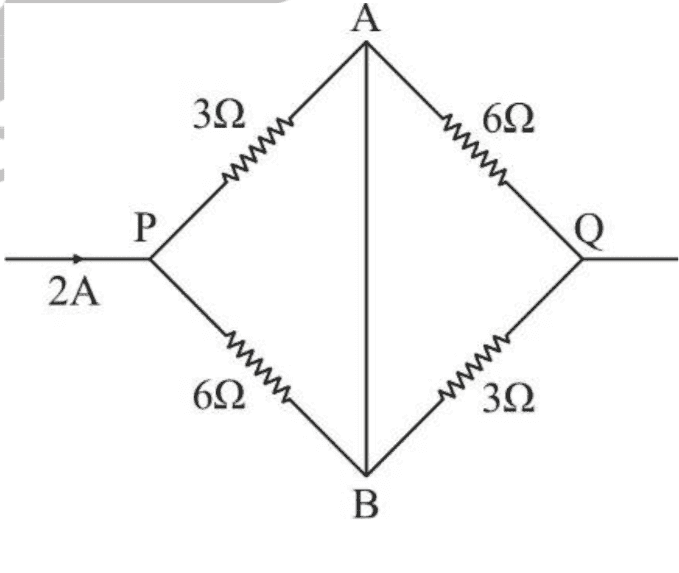# How to solve this network of resistors for equivalent resistance?

Harsharma17
Homework Statement:
The question asks to find the equivalent resistance for this group of resistors in a network.
Relevant Equations:
I used Ohm's law and parallel and series combination to solve it, but don't know where to start. I thought about using Kirchoff's laws, but still I can't find the answer.Mentor
Did you spot any resistors that are in parallel?

•Harsharma17
Homework Helper
Gold Member
Straight segment AB looks like the letter I. Would anything change if you replaced it with more straight segments that look like the letter H and tied the two resistors on the left to the left side of the H and likewise for the right side?

Remember, the convention is that, in a schematic circuit, any point on a straight line segment is at the same potential as any other point as long as there are no circuit elements, e.g. resistors, batteries, etc., in between.

•Harsharma17
Harsharma17
Straight segment AB looks like the letter I. Would anything change if you replaced it with more straight segments that look like the letter H and tied the two resistors on the left to the left side of the H and likewise for the right side?

Remember, the convention is that, in a schematic circuit, any point on a straight line segment is at the same potential as any other point as long as there are no circuit elements, e.g. resistors, batteries, etc., in between.